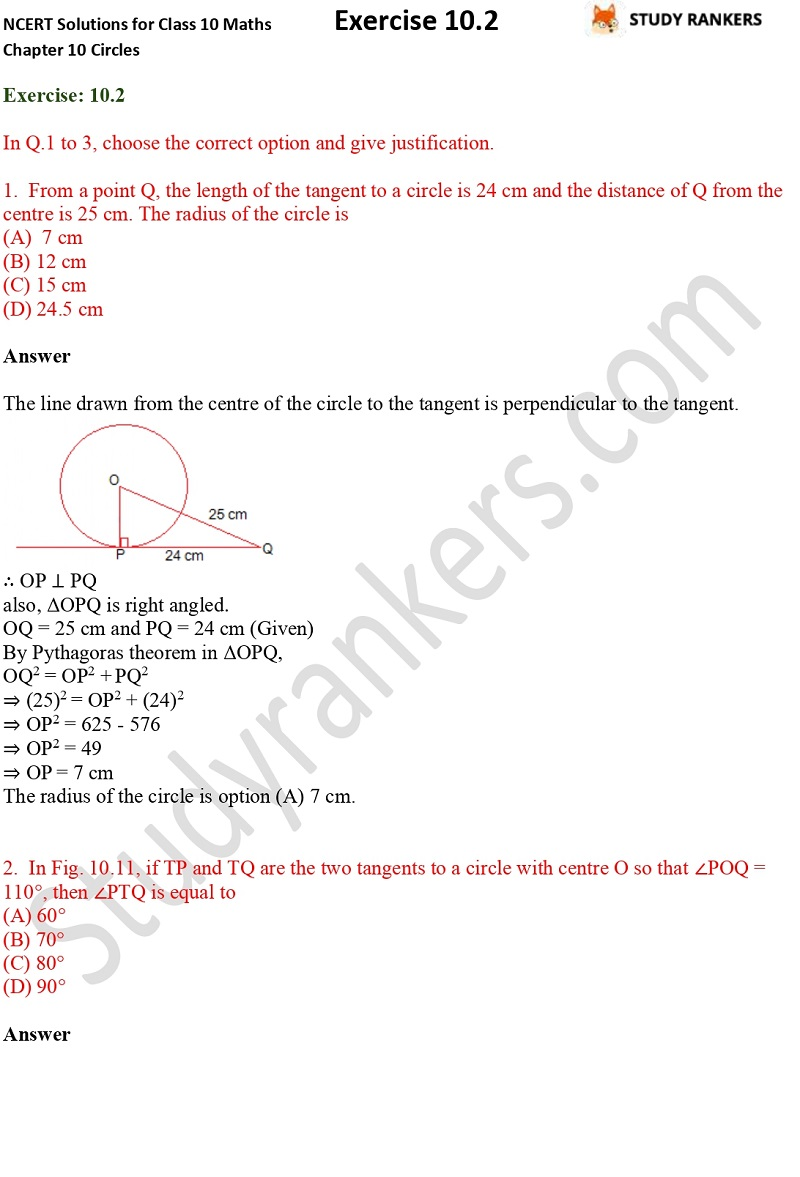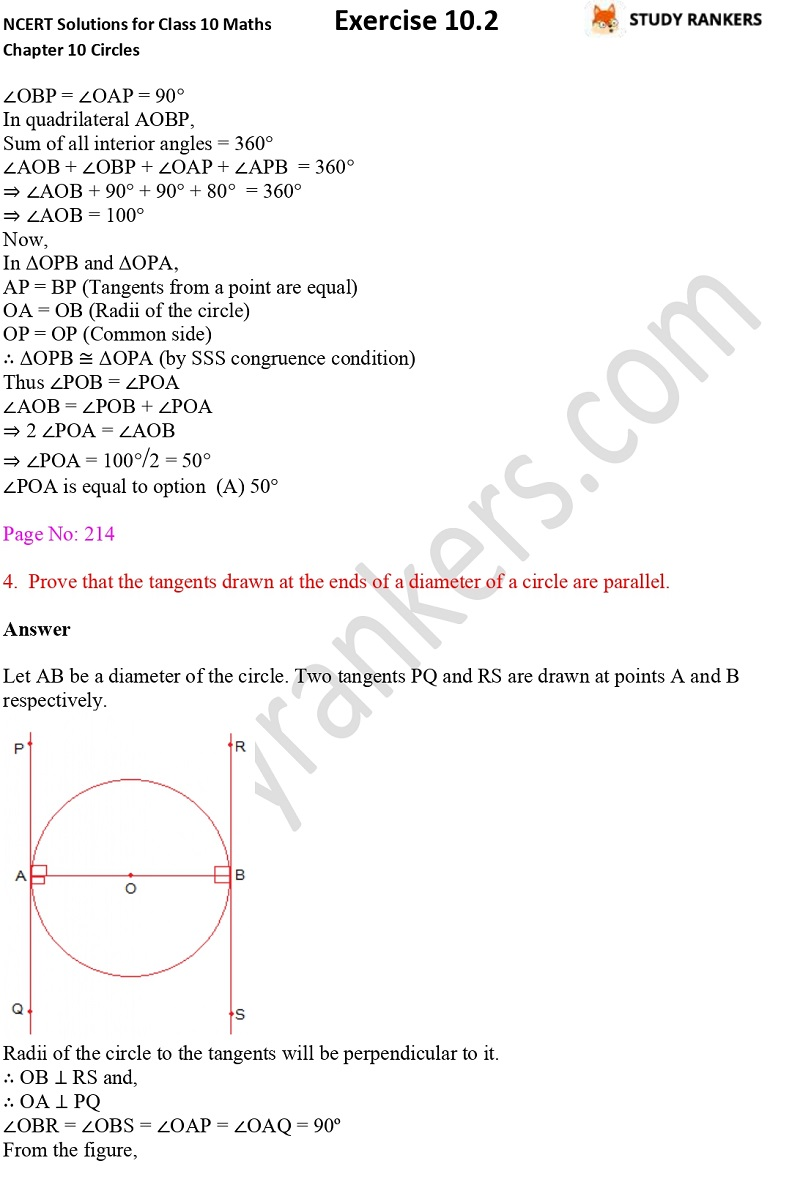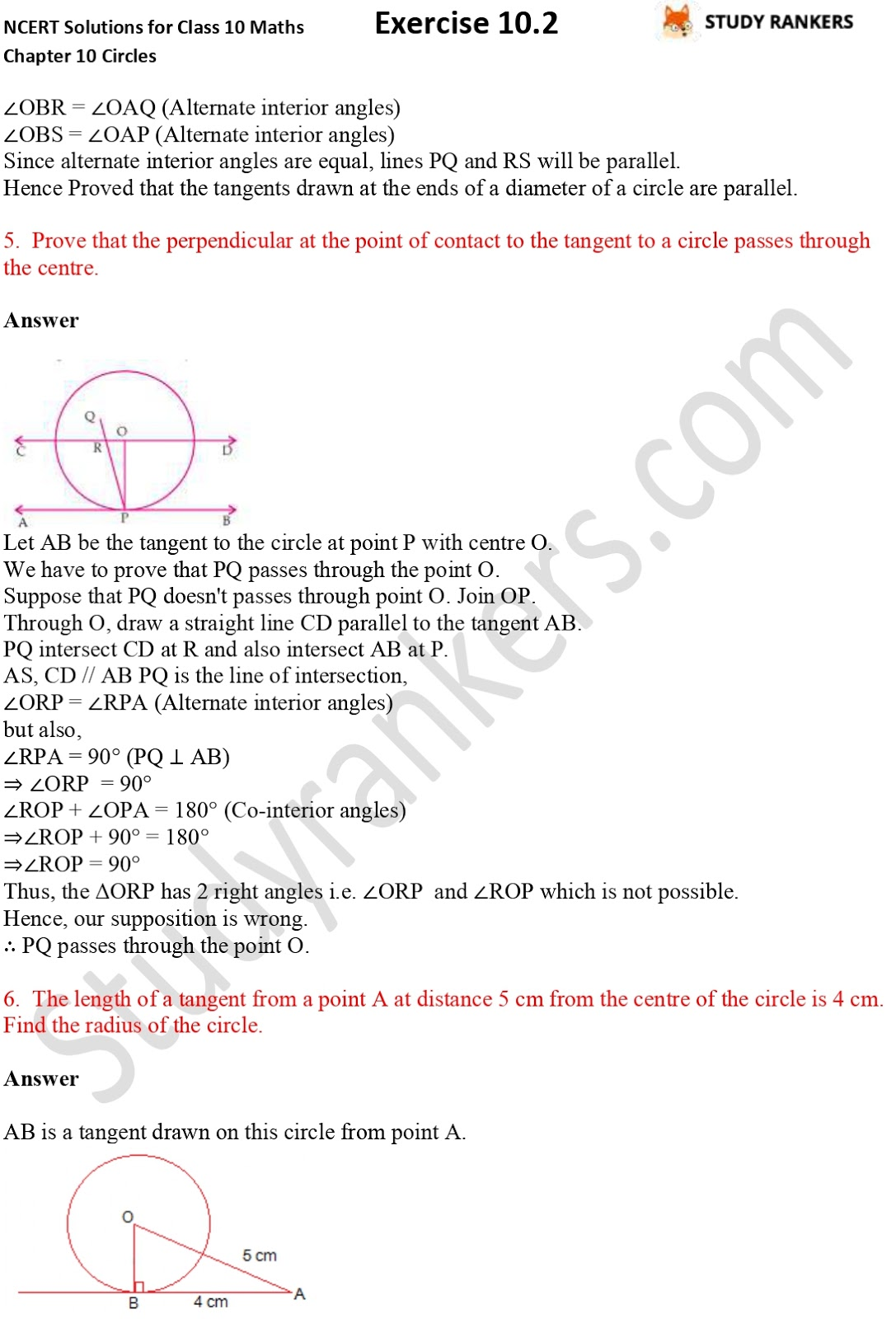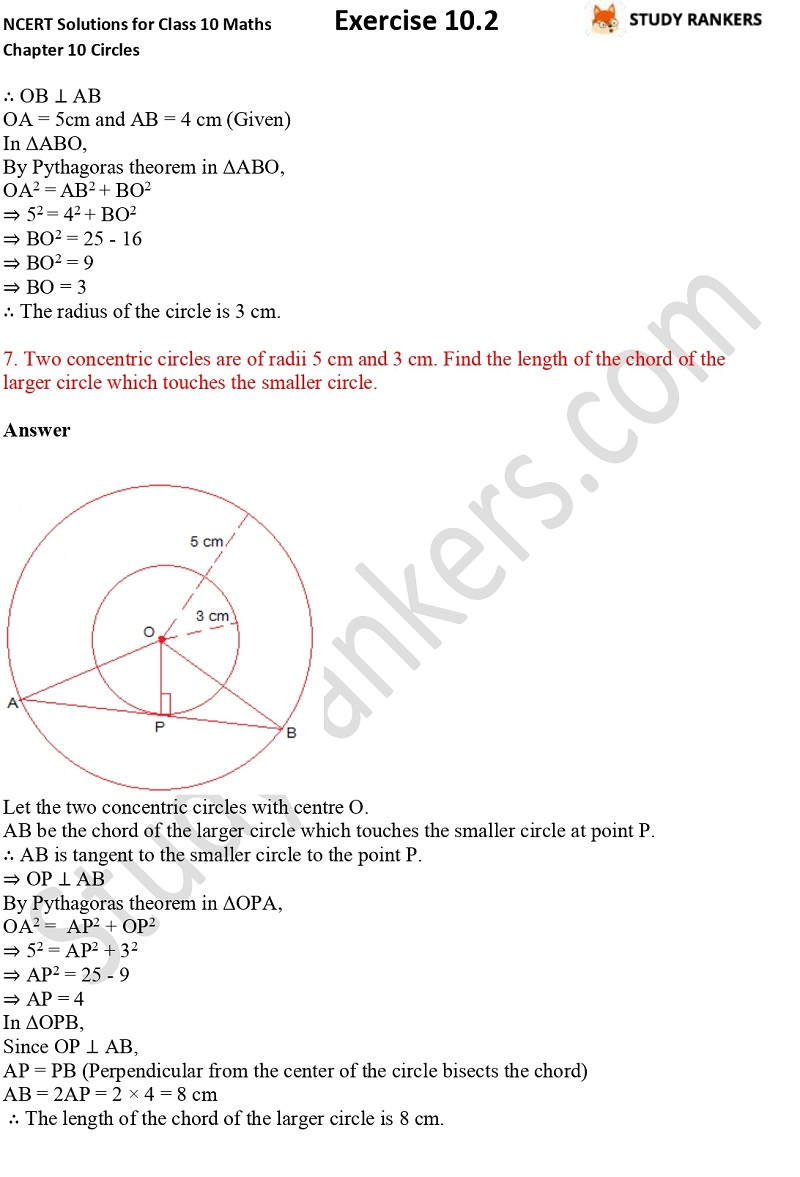>

## NCERT Solutions for Class 10 Maths Chapter 10 Circles Exercise 10.2

You will find Class 10 Maths Solutions of Chapter 10 Circles Exercise 10.2 in this page. These CBSE NCERT Solutions are prepared by Studyrankers experts that are useful in improving basic understanding of Maths and problem solving skills. These NCERT Solutions will helpful for you to score better marks in the examinations and boost your morale. We have also provided NCERT Revision notes for Class 10 through which you can memorise important points and formula given in the chapter.

There are total 13 questions in the Exercise 10.2 in which majority of the questions you have to prove a given statement such as Prove that the tangents drawn at the ends of a diameter of a circle are parallel, A quadrilateral ABCD is drawn to circumscribe a circle (see Fig. 10.12). Prove that AB + CD = AD + BC etc.X